3. Addition and subtraction of vectors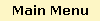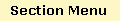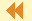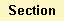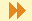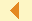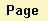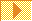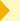GlossaryExamplesEngineering ExamplesQuizPage 7 of 7

Given a vector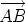and any point O, the triangle rule for addition shows us that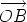=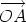+. We can rewrite this equation as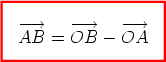Sincepoints to the head of the vectorandpoints to its tail, we call this the “head-minus-tail” rule.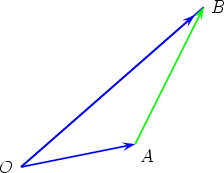The advantage of this rule is that it allows us to rewrite all the vectors occurring in a problem as combinations of vectors emanating from a given point O (often called the origin).

Feedback